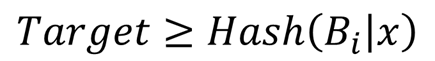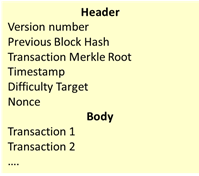# Blockchain: Proof of WorkThis post is the fourth post in a series dedicated to demystifying blockchains. The third post introduced the major concept of consensus and classified them into two categories: lottery-based and Byzantine Fault agreement-based protocols. This post presents the most known lottery-based consensus: Proof of Work (PoW). Most permissionless blockchains, such as Bitcoin or Ethereum use PoW.

The aim of a PoW algorithm is to validate a block in a distributed environment. The PoW is designed so that ill-behaved actors would need to possess the majority of the system’s computing power to validate a forged block, for instance, for implementing double spending. The PoW is information, so called the nonce in Bitcoin parlance, present inside the block to be validated. The verification of the PoW is simple and fast whereas its construction is difficult to compute. The validator must solve the cryptographic puzzle described in the first picture where

• Bi is the block to be validated.
• Target is a value determined by an authority to adjust the difficulty of the challenge. Hash is a cryptographic one-way function. One characteristic of a cryptographic one-way function is collision resistance. Hash is easy to calculate, but given a number y, it is computationally difficult to find x such as Hash(x)=y.All validators (so-called miners in the Bitcoin ecosystem) try to solve this equation. Currently, for approved cryptographic one-way functions, such as SHA-3, the only known method to solve this equation is brute force attack, i.e., exploring all the possible values systematically. The first miner to solve the equation, i.e., to find a suitable PoW, is elected the winning validator. Furthermore, the winner receives a reward (currently 25 Bitcoins). The parameter Target defines the difficulty of the challenge. Bitcoin defines the minimum number of trailing zeros that the hash must have. This number depends on the expected average solving time and the global mining computation power. In the Bitcoin ecosystem, this value is adjusted every fortnight to have an average validation time of ten minutes. If the global processing power increases too much, the difficulty target adds some zeros. Two interesting consequences:

• For a given block, there is not one unique PoW as the equation as many solutions. The winner is the first to find one solution. Two validators may find “simultaneously” a solution. The branch validation strategy addresses the corresponding problem. A future post will address branching.
• The validation time of one block is not fixed. It depends on the “luck” of the miners.

PoW works because it requires a huge calculation power. An ill-behaving actor that would control the majority of computing power would also control the system. The higher the total processing power of an entity is, the larger the probability of this entity to become the validator of a block is. With the rise of mining pools, the assumption that no entity controls a significant calculation power weakens. A mining pool is an association that federates many miners who share the reward and transaction fees. Mining pools unbalance the odds. It is not unrealistic in tiny environments that a mining pool could control more than half of the total processing power. Therefore, PoW may only be suitable for large deployments.

Finding the PoW is, by construction, computing intensive. To gain efficiency mining usually employs either Graphical Processing Units (GPU) or specialized gear based on custom developed ASICs. This calculation implies a lot of energy consumption. There is no sanctioned estimation of the total energy consumption due to mining. Nevertheless, it is commonly accepted that it is huge. Many mining farms are located in regions where energy is cheap.

PoW is surely not green nor fast.

Conclusions

PoW favors the entity that invests the most effort into validation.

PoW is a robust consensus protocol under the assumption that no entity would control more than 50% of the total mining power. At the time of Bitcoin’s creation, this assumption was valid. Currently, six mining pools have each more than 10% of the total mining power (see https://en.bitcoin.it/wiki/Comparison_of_mining_pools). This assumption may become less robust.

PoW is a huge energy consuming protocol.

## 4 thoughts on “Blockchain: Proof of Work”

1. <<>>

I think readers might get confused here, because the second sentence might be interpreted as an explanation of the first which it is not. It is just a separate statement. Collision resistance means that it is hard to find two values x and y, so that Hash(x) equals Hash(y).